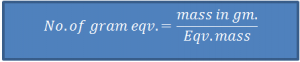# Chemistry (NMK)

Eqv. Mass of A = Mass of A

Eqv. Mass of B Mass of B

This relation tells that the combining mass reactants is always in the ratio of their equivalent mass. This law is also known as law of chemical equivalence.

• Atomic Mass = Eqv. Mass X Valency
• ‘Gram Eqv.’ or ‘Eqv.’

Eqv. mass of a substance, expressed in gram is ‘one gram equivalent’ or ‘1 eqv.’Equivalent Mass of a compound

1. Eqv. mass of acid
= Molecular Mass of acid/Basicity

Where Basicity of an acid is defined as no. of H+ ions displaced or OH- ions combined per molecule of the acid.
Eqv. mass of H2SO4 = 98 / 2 = 49

2. Eqv. mass of base
= Molecular Mass/Acidity

Where acidity of the base is defined as the no. of H+ ions consumed or OH- ions displaced per molecule of the base.
Eg.
Eq. Mass of Ca(OH)2 = 74/2 = 37

3. Eqv. mass of salt
= Molecular Mass of Salt/Total no. of charge in basic or acid radical per molecule
Eg.
Eq. mass of Na2CO3 = 106 / 2 = 53